# Ex 6.3 Squares and Square Roots- NCERT Solution Class 8

## Chapter 6 Ex.6.3 Question 1

What could be the possible one’s digits of the square root of each of the following numbers?

(i) $$9801$$

(ii) $$99856$$

(iii) $$998001$$

(iv) $$657666025$$

### Solution

What is known?

Square of numbers.

What is unknown?

Unit digit of square root.

Reasoning:

If the number ends with $$1$$, then the one’s digit of the square root of that number may be $$1$$ or $$9$$

If number ends with $$6$$, the one’s digit of the square root of that number may be $$4$$ or $$6.$$

If number ends with $$5$$, the one’s digit of square root of that number will be $$5$$. (Page $$100$$ of Grade $$8$$  NCERT Book)

Steps :

(i) $$9801$$

Since the number ends with $$1$$, the one’s digit of the square root may be $$1$$ or $$9.$$

(ii) $$99856$$

Since the number ends with $$6$$, the one’s digit of the square root may be $$4$$ or $$6.$$

(iii) $$998001$$

Since the number ends with $$1$$, the one’s digit of the square root may be $$1$$ or $$9$$.

(iv) $$657666025$$

Since the number ends with $$5,$$ the one’s digit of the square root will be $$5.$$

## Chapter 6 Ex.6.3 Question 2

Without doing any calculations, find the numbers which are surely not perfect squares.

(i) $$153$$

(ii)  $$257$$

(iii) $$408$$

(iv) $$441$$

### Solution

What is known?

Numbers

What is unknown?

Numbers which are surely not perfect square.

Reasoning:

The perfect square of a number can end with $$0, 1, 2, 4, 5, 6$$ or $$9$$ at unit’s place.

Steps

(i) $$153$$

Since the number $$153$$ has $$3$$ at its unit’s place, it is not a perfect square.

(ii) $$257$$

Since the number $$257$$ has $$7$$ at its unit’s place, it is not a perfect square.

(iii) $$408$$

Since the number $$408$$ has $$8$$ at its unit’s place, it is not a perfect square..

## Chapter 6 Ex.6.3 Question 3

Find the square roots of $$100$$ and $$169$$ by the method of repeated subtraction.

### Solution

What is known?

Perfect square

What is Unknown?

Square root of follownig square by using repeated subtraction method.

Reasoning:

The sum of the first $$n$$ odd natural numbers in$$\,{{\rm{n}}^2}$$ i.e. every square number can be expressed as a sum of successive odd numbers starting from $$1$$. (Page $$100$$ of Grade B NCERT Book)

Steps:

Consider $$\sqrt {100}$$ then

(i) $$100 – 1 = 99$$

(ii) $$99 – 3 = 96$$

(iii) $$96 – 5 = 91$$

(iv) $$91 – 7 = 84$$

(v) $$84 – 9 = 75$$

(vi) $$75 – 11 = 64$$

(vii) $$64 – 13 = 51$$

(viii) $$51 – 15 = 36$$

(ix) $$36 – 17 = 19$$

(x) $$19 – 19 = 0$$

We have subtracted successive odd numbers, and $$10$$ steps have been required for getting the result as $$0.$$

So the square root of $$100$$ is $$10$$.

Consider $$\sqrt {169}$$ , then

i) $$169 – 1= 168$$

ii)$$168 – 3= 165$$

iii) $$165 – 5=160$$

iv) $$160 – 7=153$$

v) $$153 – 9=144$$

vi)$$144 – 11=133$$

vii) $$133 – 13=120$$

viii) $$120 – 15=105$$

ix) $$105 – 17=88$$

x) $$88 – 19=69$$

xi) $$69 – 21=48$$

xii) $$48 – 23=25$$

xiii) $$25 – 25=0$$

We have subtracted successive odd numbers, and $$13$$ steps have been required for getting the result as $$0.$$

So the square root of $$169$$ is $$13$$.

## Chapter 6 Ex.6.3 Question 4

Find the square roots of the following numbers by the Prime Factorisation Method.

(i) $$729$$

(ii) $$400$$

(iii) $$1764$$

(iv) $$4096$$

(v) $$7744$$

(vi) $$9604$$

(vii) $$5929$$

(viii) $$9216$$

(ix) $$529$$

(x) $$8100$$

### Solution

What is known?

Square

What is unknown?

Squareroot by using factorisation method.

Reasoning:

As we know that each prime factor in the prime factorisation of the square of a number, occurs twice the number of times it occurs in the prime factorisation of the number itself. Let us use this to find the square root of a given square number by pairing the prime factors.

Steps:

(i)

\begin{align}729 &= 3 \times 3 \times 3 \times 3 \times 3 \times 3\\\sqrt {729} &= \sqrt {\underline {3 \times 3} \times \underline {3 \times 3} \times \underline {3 \times 3} } \\ &= 3 \times 3 \times 3\\ &= 27\end{align}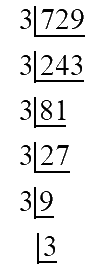ii)

\begin{align}400 &= 2 \times 2 \times 2 \times 2 \times 5 \times 5\\ \sqrt {400} &= \sqrt {\underline {2 \times 2} \times \underline {2 \times 2} \times \underline {5 \times 5} } \\&= 2 \times 2 \times 5\\&= 20\end{align}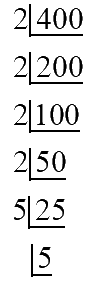ii)

\begin{align}1764 &= 2 \times 2 \times 3 \times 3 \times 7 \times 7\\\sqrt {1764} &= \sqrt {\underline {2 \times 2} \times \underline {3 \times 3} \times \underline {7 \times7}} \\&= 2 \times 3 \times 7\\&= 42\end{align}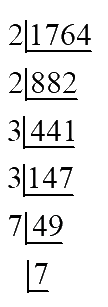iv)

\begin{align}4096 &= 2 \times 2 \times 2 \times 2 \times 2 \times 2 \times 2 \times 2 \times 2 \times 2 \times 2 \times 2\\\sqrt {4096} &= \sqrt {\underline {2 \times 2} \times \underline {2 \times 2} \times \underline {2 \times 2} \times \underline {2 \times 2} \times \underline {2 \times 2} \times \underline {2\times 2} } \\&= 2 \times 2 \times 2 \times 2 \times 2 \times 2\\&= 64\end{align}

\begin{align}&2\left| \!{\underline {\,{4096} \,}} \right. \\&2\left| \!{\underline {\,{2048} \,}} \right. \\&2\left| \!{\underline {\,{1024} \,}} \right. \\&2\left| \!{\underline {\,512 \,}} \right. \\&2\left| \!{\underline {\,256 \,}} \right.\\ &2\left| \!{\underline {\,128 \,}} \right.\\&2\left| \!{\underline {\,64\,}} \right.\\&2\left| \!{\underline {\,32 \,}} \right.\\&2\left| \!{\underline {\,16 \,}} \right.\\&2\left| \!{\underline {\,8\,}} \right.\\&2\left| \!{\underline {\,4 \,}} \right.\\ &\,\,\,\,\left| \!{\underline {\,2\,}} \right.\end{align}

(v)

\begin{align}7744 &= 2 \times 2 \times 2 \times 2 \times 2 \times 2 \times 11 \times 11\\ \sqrt {7744} &= \sqrt {\underline {2 \times 2} \times \underline {2 \times 2} \times \underline {2 \times 2} \times \underline {11 \times 11} } \\ &= 2 \times 2 \times 2 \times 11\\ &= 88\end{align}

\begin{align}&2\left| \!{\underline {\,{7744} \,}} \right. \\ &2\left| \!{\underline {\,{3872} \,}} \right. \\&2\left| \!{\underline {\,{1936} \,}} \right. \\&2\left| \!{\underline {\,968\,}} \right. \\&2\left| \!{\underline {\,484 \,}} \right.\\ &2\left| \!{\underline {\,242 \,}} \right.\\&2\left| \!{\underline {\,121\,}} \right.\\&\,\,\,\,\left| \!{\underline {\,11 \,}} \right.\end{align}

vi)

\begin{align}9604 &= 2 \times 2 \times 7 \times 7 \times 7 \times 7\\ \sqrt {9604} &= \sqrt {\underline {2 \times 2} \times \underline {7 \times 7} \times \underline {7 \times 7} } \\&= 2 \times 7 \times 7\\&= 98\end{align}

[\begin{align}&2\left| \!{\underline {\,{9604} \,}} \right. \\
&2\left| \!{\underline {\,{4802} \,}} \right. \\&7\left| \!{\underline {\,{2401} \,}} \right. \\&7\left| \!{\underline {\,343\,}} \right. \\&7\left| \!{\underline {\,49\,}} \right.\\ &\,\,\,\,\left| \!{\underline {\,7\,}} \right.\end{align}\]

(vii)

\begin{align}5929 &= 7 \times 7 \times 11 \times 11\\\sqrt {5929} &= \sqrt {\underline {7 \times 7} \times \underline {11 \times 11} } \\ &= 7 \times 11\\&= 77\end{align}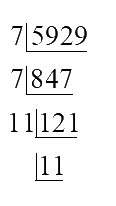iii)

\begin{align}9216 &= 2 \times 2 \times 2 \times 2 \times 2 \times 2 \times 2 \times 2 \times 2 \times 2 \times 3 \times 3\\\sqrt {9216} &= \sqrt {\underline {2 \times 2} \times \underline {2 \times 2} \times \underline {2 \times 2} \times \underline {2 \times 2} \times \underline {2 \times 2} \times \underline {3 \times 3} } \\&= 2 \times 2 \times 2 \times 2 \times 2 \times 3\\&= 96\end{align}

\begin{align}&2\left| \!{\underline {\,{9216} \,}} \right. \\&2\left| \!{\underline {\,{4608} \,}} \right. \\&2\left| \!{\underline {\,{2304} \,}} \right. \\&2\left| \!{\underline {\,1152\,}} \right. \\&2\left| \!{\underline {\,576\,}} \right.\\ &2\left| \!{\underline {\,288\,}} \right.\\&2\left| \!{\underline {\,144\,}} \right.\\&2\left| \!{\underline {\,72\,}} \right.\\&2\left| \!{\underline {\,36\,}} \right.\\&2\left| \!{\underline {\,18\,}} \right.\\&3\left| \!{\underline {\,9\,}} \right.\\&\,\,\,\,\left| \!{\underline {\,3\,}} \right.\end{align}

ix)

\begin{align}529 &= 23 \times 23\\\sqrt {529} &= \sqrt {\underline {23 \times 23} } \\ &= 23\end{align}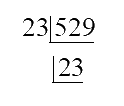x)

\begin{align}8100 &= 2 \times 2 \times 3 \times 3 \times 3 \times 3 \times 5 \times 5\\ \sqrt {8100} &= \sqrt {\underline {2 \times 2} \times \underline {3 \times 3} \times \underline {3 \times 3} \times \underline {5 \times 5} } \\& = 2 \times 3 \times 3 \times 5\\ &= 90\end{align}

\begin{align}&2\left| \!{\underline {\,{8100} \,}} \right. \\&2\left| \!{\underline {\,{4050} \,}} \right. \\&3\left| \!{\underline {\,{2025} \,}} \right. \\&3\left| \!{\underline {\,675\,}} \right. \\&3\left| \!{\underline {\,225\,}} \right.\\ &3\left| \!{\underline {\,75\,}} \right.\\&5\left| \!{\underline {\,25\,}} \right.\\&\,\,\,\,\left| \!{\underline {\,5\,}} \right.\end{align}

## Chapter 6 Ex.6.3 Question 5

For each of the following numbers, find the smallest whole number by which it should be multiplied so as to get a perfect square number. Also find the square root of the square number so obtained.

(i) $$252$$

(ii) $$180$$

(iii) $$1008$$

(iv) $$2028$$

(v) $$1458$$

(vi) $$768$$

### Solution

What is known?

Numbers

What is unknown?

The smallest whole number by which it should be multiplied so as to get a perfect square number

Reasoning:

To get a perfect square, each factor of the given number must be paired.

Steps:

(i) $$252$$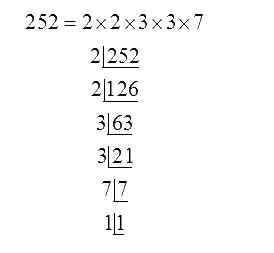Hence, Prime factor $$7$$ does not have its pair. If $$7$$ gets a pair, then the number becomes a perfect square. Therefore, $$252$$ has to be multiplied with $$7$$ to get a perfect square.

So, perfect square is $$252 \times 7 = 1764$$

$$1764 \! = \! 2 \! \times \! 2 \! \times \! 3 \! \times \! 3 \! \times \! 7 \! \times \! 7$$

So, perfect root of $$1764$$ is $$2 \times 3 \times 7 = 42$$

(ii) $$180$$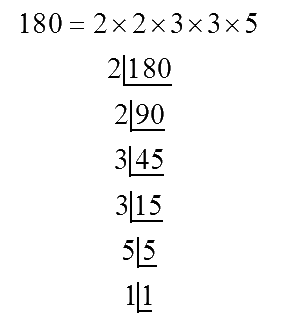Hence, prime factor $$5$$ does not have its pair. If $$5$$ gets a pair, then the number becomes a perfect square. Therefore, $$180$$ has to be multiplied with $$5$$ to get a perfect square.

So, perfect square is $$180 \times 5 = 900$$

$$900 \! = \! 2 \! \times \! 2 \! \times \! 3 \! \times \! 3 \! \times \! 5 \! \times \! 5$$

So, perfect root of $$900$$ is $$2 \times 3 \times 5 = 30$$

(iii) $$1008$$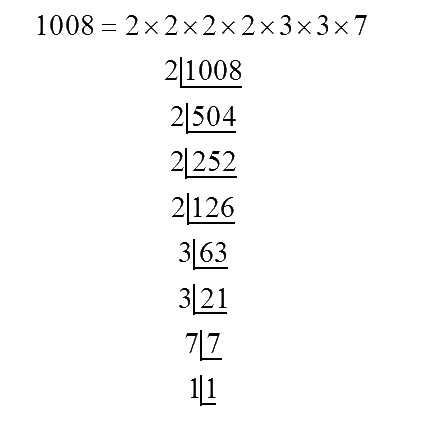Hence, prime factor $$7$$ does not have its pair. If $$7$$ gets a pair, then the number becomes a perfect square. Therefore, $$1008$$ has to be multiplied with $$7$$ to get a perfect square.

So, perfect square is $$1008 \times 7 = 7056$$

$$7056 \! = \! 2 \! \times \! 2 \! \times \! 2 \! \times \! 2 \! \times \! 3 \! \times \! 3 \! \times \! 7 \! \times \! 7$$

So, perfect root of

$$7056$$ is $$2 \times 2 \times 3 \times 7= 84$$

(iv) $$2028$$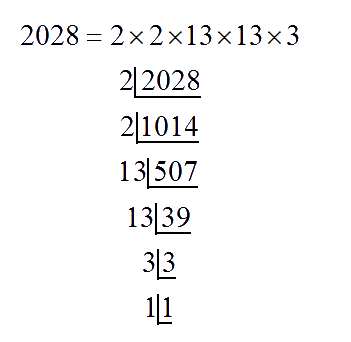Hence, prime factor $$3$$ does not have its pair. If $$3$$ gets a pair, then the number becomes a perfect square. Therefore, $$2028$$ has to be multiplied with $$3$$ to get a perfect square.

So, perfect square is $$2028 \times 3 = 6084$$

$$6084 \! = \! 2 \! \times \! 2 \! \times \! 13 \! \times \! 13 \! \times \! 3 \! \times \! 3$$

So, perfect root of $$6084$$ is $$2 \times 13 \times 3 = 78$$

(v) $$1458$$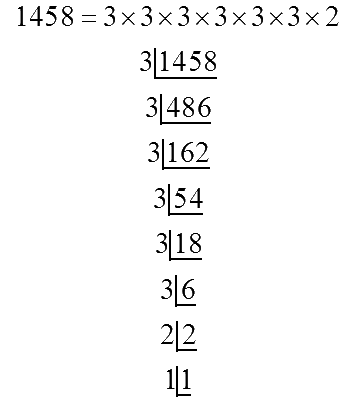Hence, prime factor $$2$$ does not have its pair. If $$2$$ gets a pair, then the number becomes a perfect square. Therefore, $$1458$$ has to be multiplied with $$2$$ to get a perfect square.

So, perfect square is $$1458 \times 2 = 2916$$

$$2916 \! = \! 3 \! \times \! 3 \! \times \! 3 \! \times \! 3 \! \times \! 3 \! \times \! 3 \! \times \! 2 \! \times \! 2$$

So, perfect root of $$2916$$ is $$3 \! \times \! 3 \! \times \! 3 \! \times \! 2 \! = \! 54$$

(vi) $$768$$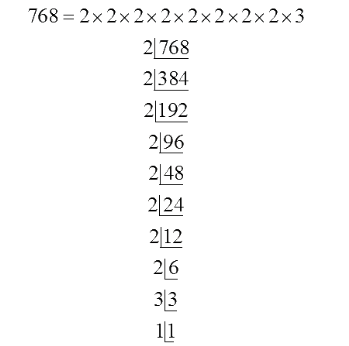Hence, prime factor $$3$$ does not have its pair. If $$3$$ gets a pair, then the number becomes a perfect square. Therefore, $$768$$ has to be multiplied with $$3$$ to get a perfect square.

So, perfect square is $$768 \times 3 = 2304$$

$$2304 \! = \! 2 \! \times \! 2 \! \times \! 2 \! \times \! 2 \! \times \! 2 \! \times \! 2 \! \times \! 2 \! \times \! 2 \! \times \! 3 \! \times \! 3$$

So, perfect root of $$2304$$ is $$2 \! \times \! 2 \! \times \! 2 \! \times \! 2 \! \times \! 3 \! = \! 48$$

## Chapter 6 Ex.6.3 Question 6

For each of the following numbers, find the smallest whole number by which it should be divided so as to get a perfect square. Also find the square root of the square number so obtained.

(i) $$252$$

(ii) $$2925$$

(iii) $$396$$

(iv) $$2645$$

(v) $$2800$$

(vi)$$1620$$

### Solution

What is known?

Numbers

What is unknown?

The smallest whole number by which it should be divided so as to get a perfect square number.

Reasoning:

To get a perfect square, each factor of the given number must be paired.

Steps:

(i) $$252$$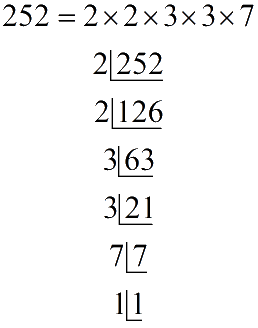Hence, prime factor $$7$$ does not have its pair. If number is divided by $$7$$ then rest of the prime factor will be in pairs.

Therefore, $$252$$ has to be divided by $$7$$ to get a perfect square.

$252 \div 7 = 36$

$$36$$ is perfect square

\begin{align}36 &= 2 \times 2 \times 3 \times 3\\ &= {2^2} \times {3^2}\\ &= {(2 \times 3)^2}\\\sqrt {36} &= 2 \times 3 = 6\end{align}

(ii) $$2925$$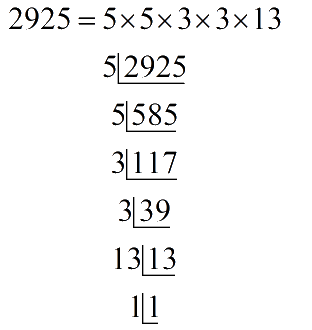Hence, prime factor $$13$$ does not have its pair. If number is divided by $$13$$ then rest of the prime factor will be in pairs.

Therefore, $$2925$$ has to be divided by $$13$$ to get a perfect square.

$2925 \div 13 = 225$

$$225$$ is a perfect square

\begin{align}225 &= 5 \times 5 \times 3 \times 3\\&= {5^2} \times {3^2}\\&= {(5 \times 3)^2}\\\sqrt {225}&= 15\end{align}

(iii) $$396$$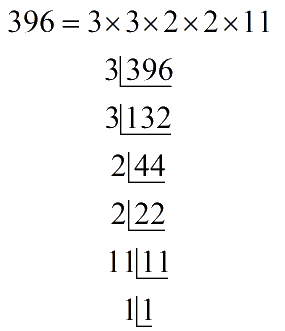Hence, prime factor $$11$$ does not have its pair. If number is divided by $$11$$ then rest of the prime factor will be in pairs. Therefore, $$396$$ has to be divided by $$11$$ to get a perfect square.

\begin{align} 396 \div 11 &= 36\\ 36 &= 3 \times 3 \times 2 \times 2\\&= {3^2} \times {2^2}\\&= {(3 \times 2)^2}\\\sqrt {36}&= 3 \times 2 = 6\end{align}

$$36$$ isa perfect square

(iv) $$2645$$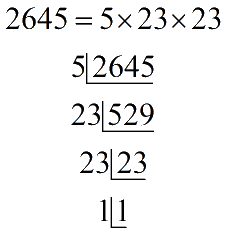Hence, prime factor $$5$$ does not have its pair. If number is divided by $$5$$ then rest of the prime factor will be in pairs. Therefore, $$2645$$ has to be divided by $$5$$ to get a perfect square.

$2645 \div 5 = 529$

$$592$$ is a perfect square

\begin{align}529 &= 23 \times 23\\&= {23^2}\\\sqrt {529}&= 23\end{align}

(v) $$2800$$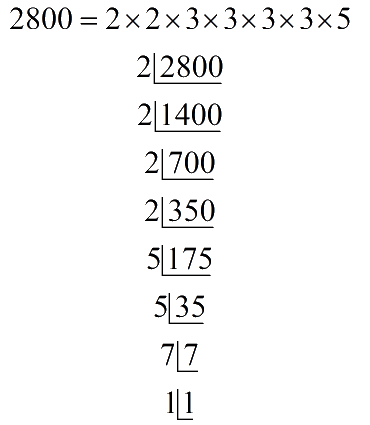Hence, prime factor $$7$$ does not have its pair. If number is divided by $$7$$ then rest of the prime factor will be in pairs. Therefore, $$2800$$ has to be divided by $$7$$ to get a perfect square

$2800 \div 7 = 400$

$$400$$ is a perfect square

\begin{align}400 &= 2 \times 2 \times 2 \times 2 \times 5 \times 5\\ &= {2^2} \times {2^2} \times {5^2}\\ &= {(2 \times 3 \times 5)^2}\\\sqrt {400} &= 2 \times 2 \times 5\\ &= 20\end{align}

(vi) $$1620$$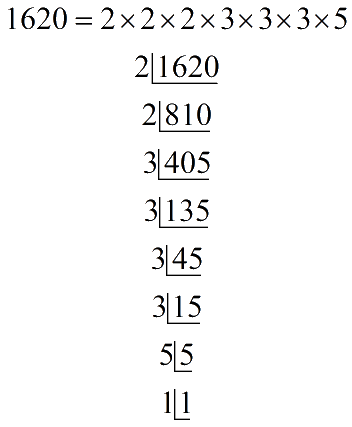Hence, prime factor $$5$$ does not have its pair. If number is divided by $$5$$ then rest of the prime factor will be in pairs. Therefore, $$1620$$ has to be divided by $$5$$ to get a perfect square.

$1620 \div 5 = 324$

$$324$$ is a perfect square

\begin{align}324 &= 2 \times 2 \times 3 \times 3 \times 3 \times 3\\&= {2^2} \times {3^2} \times {3^2}\\ &= {(2 \times 3 \times 3)^2}\\\sqrt {324}&= 2 \times 3 \times 3\\&= 18\end{align}

## Chapter 6 Ex.6.3 Question 7

The students of Class $$VIII$$ of a school donated $$\rm Rs. 2401$$ in all for the Prime Minister’s National Relief Fund. Each student donated as many rupees as the number of students in the class. Find the number of students in the class.

### Solution

What is Known?

Total amount of donation donated by students.

What is Unknown?

Number of students in the class.

Reasoning:

Each student donated as many rupees as the number of students in the class i.e. money donated by each student is equal to the number of students in the class. Total amount of donation is equal to the product of number of students in the class and money donated by each student.

Steps:

Let number of students in a class be $$x$$

So, money donated by each student is also $$x$$ (as they donated as much amount as number of students in the class).

Total amount of donation $$= \rm Rs \,2401$$

Total amount of donation $$=$$ number of students $$\times$$money donated by each student

\begin{align}2401 &= x \times x\\2401 &= {x^2}\\x &= \sqrt {2401} \end{align}

\begin{align}&7\left| \!{\underline {\,{2401} \,}} \right. \\&7\left| \!{\underline {\,{343} \,}} \right. \\&7\left| \!{\underline {\,{49} \,}} \right. \\&7\left| \!{\underline {\,7 \,}} \right. \\&1\left| \!{\underline {\,1 \,}} \right. \end{align}

\begin{align}&x = \sqrt {2401} \\&x = \sqrt {7 \times 7 \times 7 \times 7} \\&x = \sqrt {{7^2} \times {7^2}} \\&x = \sqrt {{{(7 \times 7)}^2}} \\&x = 7 \times 7 = 49\end{align}

Therefore, the number of students in class is $$49$$.

## Chapter 6 Ex.6.3 Question 8

$$2025$$ plants are to be planted in a garden in such a way that each row contains as many plants as the number of rows. Find the number of rows and the number of plants in each row.

### Solution

What is known?

Number of plants that is to be planted in the garden.

What is Unknown?

Number of rows and the number of plants in each row.

Reasoning:

Each row contains as many plants as the number of rows i.e. number of rows equal to number of plants in each row. Total number of plants that to be planted is equal to the product of number of plants and number of rows.

Steps:

Let number of rows be $$x$$

Number of plants in each row is also $$x$$

(number of rows) (number of plants in each row)  $$=2025$$

\begin{align}x \times x &= 2025\\{x^2} &= 2025\\x &= \sqrt {2025} \end{align}

\begin{align}&5\left| \!{\underline {\,{2025} \,}} \right. \\&5\left| \!{\underline {\,{405} \,}} \right. \\&3\left| \!{\underline {\,{81} \,}} \right. \\&3\left| \!{\underline {\,{27} \,}} \right. \\&3\left| \!{\underline {\,9 \,}} \right. \\&3\left| \!{\underline {\,3 \,}} \right. \\&1\left| \!{\underline {\,1 \,}} \right. \end{align}

\begin{align}x &= \sqrt {2025} \\x &= \sqrt {5 \times 5 \times 3 \times 3 \times 3 \times 3} \\x &= \sqrt {{5^2} \times {3^2} \times {3^2}} \\x &= \sqrt {{{(5 \times 3 \times 3)}^2}} \\x &= 5 \times 3 \times 3 = 45\end{align}

Therefore, the number of rows and the number of plants in each row is $$45$$.

## Chapter 6 Ex.6.3 Question 9

Find the smallest square number that is divisible by each of the numbers $$4$$,$$9$$ and $$10$$.

### Solution

What is known?

Divisor are 4, 9 and 10

What is unknown?

The smallest square that is divisible by 4, 9 and 10.

Reasoning:

The number that will be perfectly divisible by each one of the numbers is their $$LCM$$.

Steps:

\begin{align}&2\left| \!{\underline {\,{4,9,10} \,}} \right. \\&2\left| \!{\underline {\,{2,9,5} \,}} \right. \\&3\left| \!{\underline {\,{1,9,5} \,}} \right. \\&3\left| \!{\underline {\,{1,3,5} \,}} \right. \\&5\left| \!{\underline {\,{1,1,5} \,}} \right. \\&1\left| \!{\underline {\,{1,1,1} \,}} \right. \end{align}

$$LCM$$ of $$4,9,10$$
\begin{align}& =\text{ }2\times ~2\times 3\times 3~\times 5 \\& =\text{ }180 \\ \end{align}

Hence, number $$5$$ does not have a pair. Therefore $$180$$ is multiplied by $$5$$ then the number obtained is a perfect square. Therefore, $$180~\times 5\text{ }=\text{ }900$$

So, $$900$$ is the smallest square that is divisible by $$4$$, $$9$$ and $$10$$.

## Chapter 6 Ex.6.3 Question 10

Find the smallest square number that is divisible by each of the numbers $$8$$, $$15$$ and $$20$$

### Solution

What is known?

Divisor are $$8, 15$$ and $$20$$

What is unknown?

The smallest square that is divisible by $$8, 15$$ and $$20$$

Reasoning:

The number that will be perfectly divisible by each one of the numbers is their $$LCM$$.

Steps:

$${\rm{LCM \,of }}\,8,15,20$$

\begin{align}&2\left|\!{\underline {\,{8,15,20} \,}} \right. \\&2\left| \!{\underline {\,{4,15,10} \,}} \right. \\&2\left| \!{\underline {\,{2,15,5} \,}} \right. \\&3\left| \!{\underline {\,{1,15,5} \,}} \right. \\&5\left| \!{\underline {\,{1,5,5} \,}} \right. \\&1\left| \!{\underline {\,{1,1,1} \,}} \right. \\\end{align}

$$LCM$$ of $$8,15,20$$
\begin{align} &= 2 \times 2 \times 2\times 3 \times 5 \\&=120\end{align}

Hence prime factor $$2, 3, 5$$ do not have their respective pairs. Therefore $$120$$ is not a perfect square. If $$120$$ is multiplied by $$2 \times 3 \times 5$$ then the number obtained will be a perfect square.

Therefore, $$120 \times 2 \times 3 \times 5 = 3600$$

Thus, the perfect square number is $$3600$$ which is completely divisible by $$8, 15$$ and $$20.$$

Instant doubt clearing with Cuemath Advanced Math Program